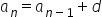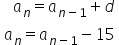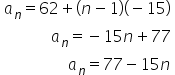Maths-
General
Easy

Question

# Write recursive formula and explicit formula. 62,57,52,47,42,...Hint:

## The correct answer is: a_n=77-15n

### Explanation: We have given a sequence 62,57,52,47,42,.... We have to find the recursive and explicit formula of the given sequence. Step 1 of 2:We have given a sequence 62,57,52,47,42,....The given sequence is an AP.We know that the recursive formula for any AP is, where d is common difference.Here the common difference is -15So, The recursive formula isStep 2 of 2:The given sequence is an AP.So, The explicit formula will be#### With Turito Foundation.#### Get an Expert Advice From Turito.